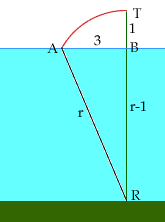Quandaries and Queries Hi:  Can you help me with these problems: 1) A water lily with a rigid stem extends one foot above the surface of the water.  When pulled over, it disappears beneath the water at a distance 3 feet from where the stem originally entered the water.  How deep is the pond? Thanks Inba Hi Inba, I drew a diagram.T is the top if the water lily, R is the point where the stem enters the bottom of the pond, B is the point on the surface of the water where the stem breaks through the surface, and A is the point where the pulled over plant disappears below the surface. AT is an arc of a circle with radius r = |AR|, |AB| = 3 and |BT| = 1 so |BR| = r-1. The triangle ARB has a right angle at B and thus you can use Pythagoras' Theorem to write and equation which you can solve for r. Penny Go to Math Central